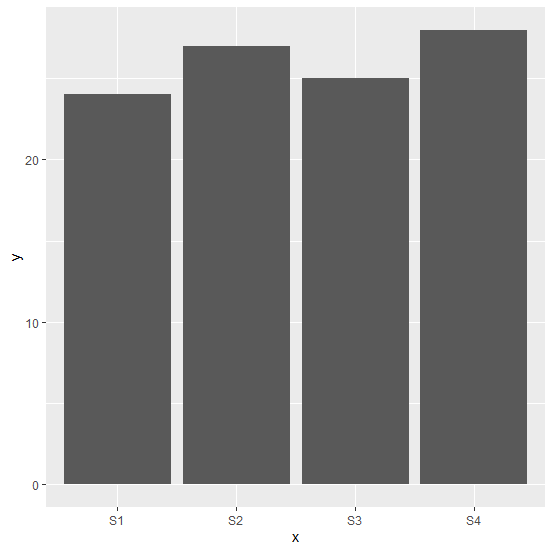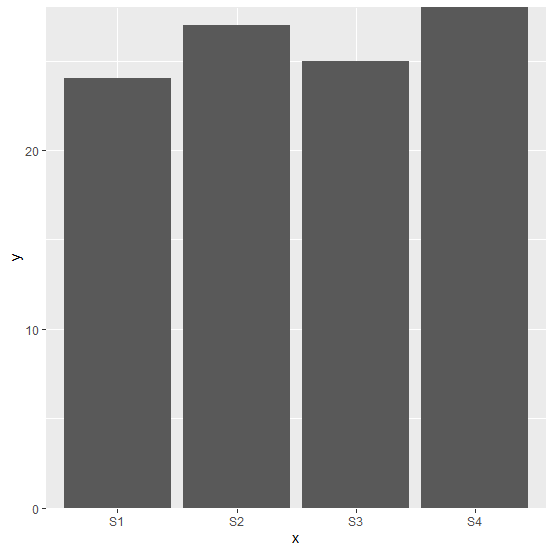# How to align the bars of a barplot with the X-axis using ggplot2 in R?

The bar plot is created with geom_bar function but there always exist some space between the bars and the X-axis labels. If we want to reduce that space or completely remove it we need to use scale_y_continuous function by defining expand argument for former and scale_y_continuous(expand=c(0,0)) for latter.

## Example

Live Demo

Consider the below data frame −

set.seed(888)
x<-c("S1","S2","S3","S4")
y<-c(24,27,25,28)
df<-data.frame(x,y)
df

## Output

   x y
1 S1 24
2 S2 27
3 S3 25
4 S4 28

library(ggplot2)
ggplot(df,aes(x,y))+geom_bar(stat="identity")

## OutputCreating the bar plot without space between X-axis labels and the bars −

ggplot(df,aes(x,y))+geom_bar(stat="identity")+scale_y_continuous(expand=c(0,0))

## Output